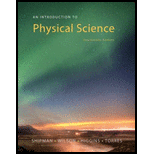Chapter 1, Problem 5MCAn Introduction to Physical Science

14th Edition
James Shipman + 3 others
ISBN: 9781305079137

Solutions

Chapter
SectionAn Introduction to Physical Science

14th Edition
James Shipman + 3 others
ISBN: 9781305079137
Textbook Problem

Which of the following is not a fundamental quantity? (a) length (b) weight (c) mass (d) time

To determine
Check in which options is not a fundamental quantity.

Explanation

Given Info: Fundamental quantities are given.

Explanation:

A fundamental quantity does not change at different locations. Mass and weight are related, but they are not the same. Mass, length and time are fundamental quantities and weight is not the fundamental quantity...

Still sussing out bartleby?

Check out a sample textbook solution.

See a sample solution

The Solution to Your Study Problems

Bartleby provides explanations to thousands of textbook problems written by our experts, many with advanced degrees!

Get Started

Find more solutions based on key concepts